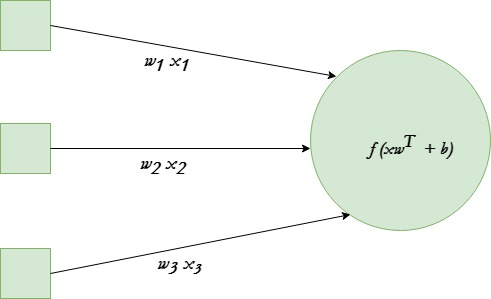Skip to content
Related Articles
A single neuron neural network in Python
• Difficulty Level : Hard
• Last Updated : 11 Jun, 2021

Neural networks are the core of deep learning, a field which has practical applications in many different areas. Today neural networks are used for image classification, speech recognition, object detection etc. Now, Let’s try to understand the basic unit behind all this state of art technique.
A single neuron transforms given input into some output. Depending on the given input and weights assigned to each input, decide whether the neuron fired or not. Let’s assume the neuron has 3 input connections and one output.We will be using tanh activation function in given example.
The end goal is to find the optimal set of weights for this neuron which produces correct results. Do this by training the neuron with several different training examples. At each step calculate the error in the output of neuron, and back propagate the gradients. The step of calculating the output of neuron is called forward propagation while calculation of gradients is called back propagation.
Below is the implementation :

## Python3

 `# Python program to implement a``# single neuron neural network` `# import all necessary libraries``from` `numpy ``import` `exp, array, random, dot, tanh` `# Class to create a neural``# network with single neuron``class` `NeuralNetwork():``    ` `    ``def` `__init__(``self``):``        ` `        ``# Using seed to make sure it'll ``        ``# generate same weights in every run``        ``random.seed(``1``)``        ` `        ``# 3x1 Weight matrix``        ``self``.weight_matrix ``=` `2` `*` `random.random((``3``, ``1``)) ``-` `1` `    ``# tanh as activation function``    ``def` `tanh(``self``, x):``        ``return` `tanh(x)` `    ``# derivative of tanh function.``    ``# Needed to calculate the gradients.``    ``def` `tanh_derivative(``self``, x):``        ``return` `1.0` `-` `tanh(x) ``*``*` `2` `    ``# forward propagation``    ``def` `forward_propagation(``self``, inputs):``        ``return` `self``.tanh(dot(inputs, ``self``.weight_matrix))``    ` `    ``# training the neural network.``    ``def` `train(``self``, train_inputs, train_outputs,``                            ``num_train_iterations):``                                ` `        ``# Number of iterations we want to``        ``# perform for this set of input.``        ``for` `iteration ``in` `range``(num_train_iterations):``            ``output ``=` `self``.forward_propagation(train_inputs)` `            ``# Calculate the error in the output.``            ``error ``=` `train_outputs ``-` `output` `            ``# multiply the error by input and then``            ``# by gradient of tanh function to calculate``            ``# the adjustment needs to be made in weights``            ``adjustment ``=` `dot(train_inputs.T, error ``*``                             ``self``.tanh_derivative(output))``                             ` `            ``# Adjust the weight matrix``            ``self``.weight_matrix ``+``=` `adjustment` `# Driver Code``if` `__name__ ``=``=` `"__main__"``:``    ` `    ``neural_network ``=` `NeuralNetwork()``    ` `    ``print` `(``'Random weights at the start of training'``)``    ``print` `(neural_network.weight_matrix)` `    ``train_inputs ``=` `array([[``0``, ``0``, ``1``], [``1``, ``1``, ``1``], [``1``, ``0``, ``1``], [``0``, ``1``, ``1``]])``    ``train_outputs ``=` `array([[``0``, ``1``, ``1``, ``0``]]).T` `    ``neural_network.train(train_inputs, train_outputs, ``10000``)` `    ``print` `(``'New weights after training'``)``    ``print` `(neural_network.weight_matrix)` `    ``# Test the neural network with a new situation.``    ``print` `(``"Testing network on new examples ->"``)``    ``print` `(neural_network.forward_propagation(array([``1``, ``0``, ``0``])))`

Output :

```Random weights at the start of training
[[-0.16595599]
[ 0.44064899]
[-0.99977125]]

New weights after training
[[5.39428067]
[0.19482422]
[0.34317086]]

Testing network on new examples ->
[0.99995873]```

Attention geek! Strengthen your foundations with the Python Programming Foundation Course and learn the basics.

To begin with, your interview preparations Enhance your Data Structures concepts with the Python DS Course. And to begin with your Machine Learning Journey, join the Machine Learning – Basic Level Course

My Personal Notes arrow_drop_up